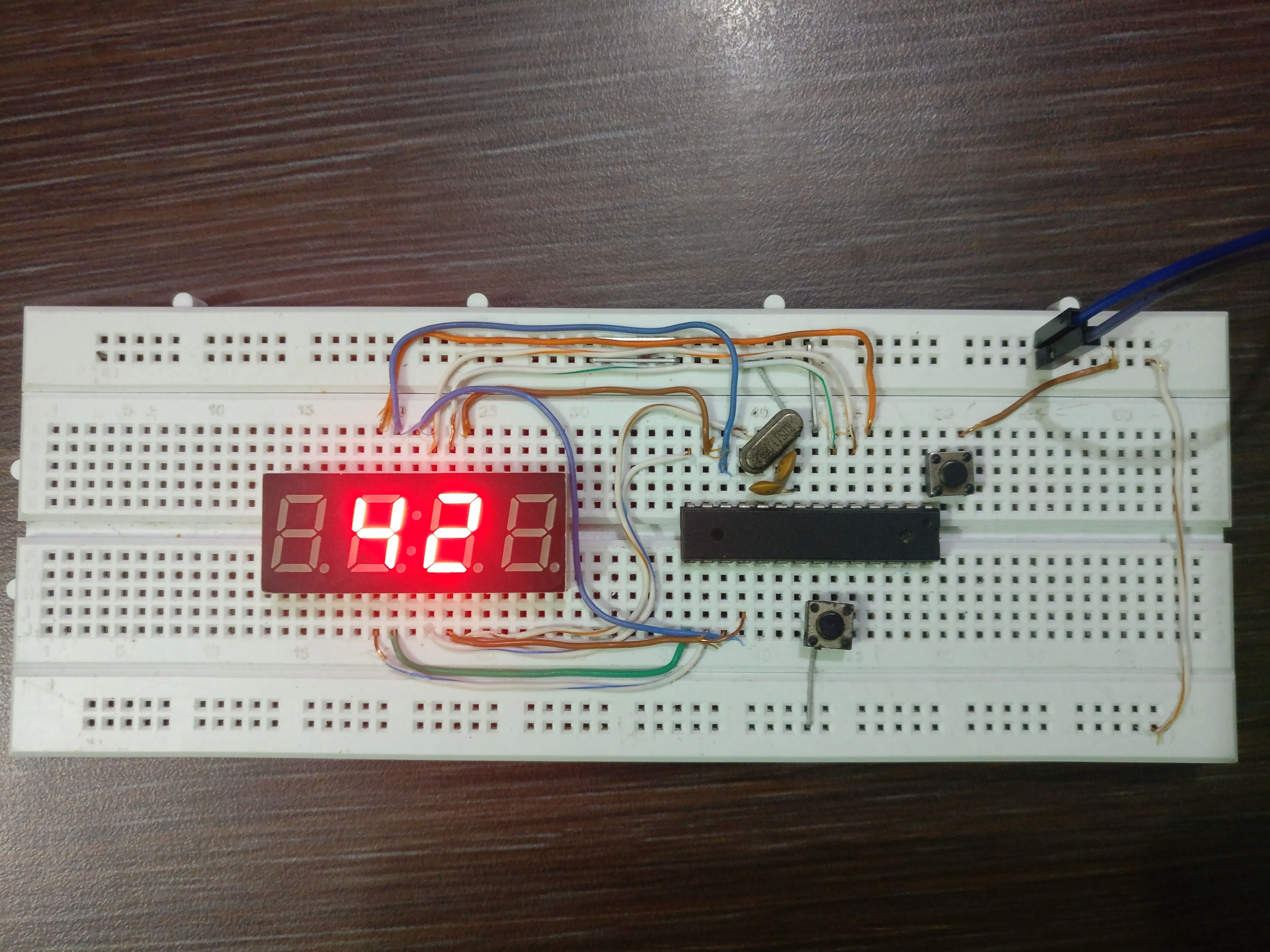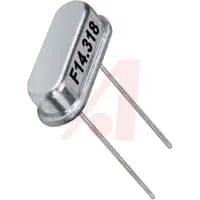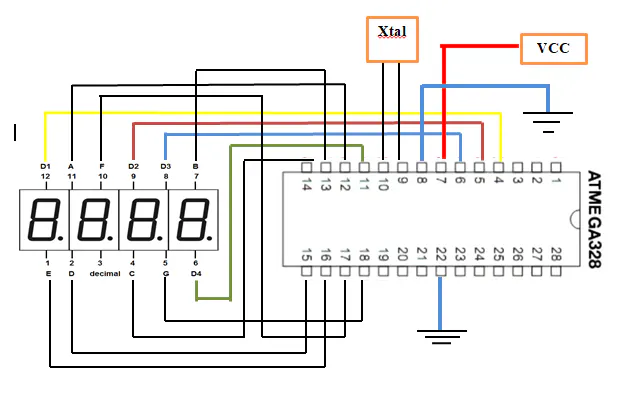# Two Digits (00-99) Up and Down Counter

The final product of this tutorial is a two digit up-down counter using ATmega328 microcontroller.

IntermediateFull instructions provided2 hours2,242## Things used in this project

### Hardware componentsCapacitor 220 µF
×2×116 MHz Crystal
×1Jumper wires (generic)
×1

### Software apps and online servicesArduino IDE

## Schematics

### Schematic Design## Code

### Arduino Code

Arduino
Two Digit Up-Down Counter using Atmega328 Microcontroller
```int a = 6, b = 7, c = 8, d = 9, e = 10, f = 11, g = 12, dp = 13; //Display pins
int d1 = 5, d2 = 4, d3 = 3, d4 = 2; //Common pins
int time = 50; //speed of the counter in ms
int buttonPin = 14;
int val = 0;
void setup() {
int i = 1;
for(; i<13; i++)
pinMode(i, OUTPUT);
pinMode(buttonPin, INPUT_PULLUP) }
void loop() {
offd(d1);
offd(d2);
offd(d3);
offd(d4);
int i, j;
if ( val == HIGH ){ //The counter
for(i = 0; i<1000; i++) {
for(j = 0; j <= (time/20); j++)  {
ond(d2);
num(i/10 - 10*(i/100));
delay(5);
offd(d2);
ond(d3);
num(i/100 - 10*(i/1000));
delay(5);
offd(d3); }  } }
if ( val == LOW )
{   //The counter
for(i = 1000; i>=0; i--)
{  for(j = 0; j <= (time/20); j++) {
ond(d2);
num(i/10 - 10*(i/100));
delay(5);
offd(d2);
ond(d3);
num(i/100 - 10*(i/1000));
delay(5);
offd(d3);  } } } }
void on(int i) {
digitalWrite(i, HIGH);}
void off(int i){
digitalWrite(i, LOW); }
void ond(int i) {
digitalWrite(i, LOW);}
void offd(int i){
digitalWrite(i, HIGH); }
void num(int n) {
if(n < 0)
n = 0;
switch(n) {
case 0:
on(a);
on(b);
on(c);
on(d);
on(e);
on(f);
off(g);
break;
case 1:
off(a);
on(b);
on(c);
off(d);
off(e);
off(f);
off(g);
break;
case 2:
on(a);
on(b);
off(c);
on(d);
on(e);
off(f);
on(g);
break;
case 3:
on(a);
on(b);
on(c);
on(d);
off(e);
off(f);
on(g);
break;
case 4:
off(a);
on(b);
on(c);
off(d);
off(e);
on(f);
on(g);
break;
case 5:
on(a);
off(b);
on(c);
on(d);
off(e);
on(f);
on(g);
break;
case 6:
on(a);
off(b);
on(c);
on(d);
on(e);
on(f);
on(g);
break;
case 7:
on(a);
on(b);
on(c);
off(d);
off(e);
off(f);
off(g);
break;
case 8:
on(a);
on(b);
on(c);
on(d);
on(e);
on(f);
on(g);
break;
case 9:
on(a);
on(b);
on(c);
on(d);
off(e);
on(f);
on(g);
break;}
```